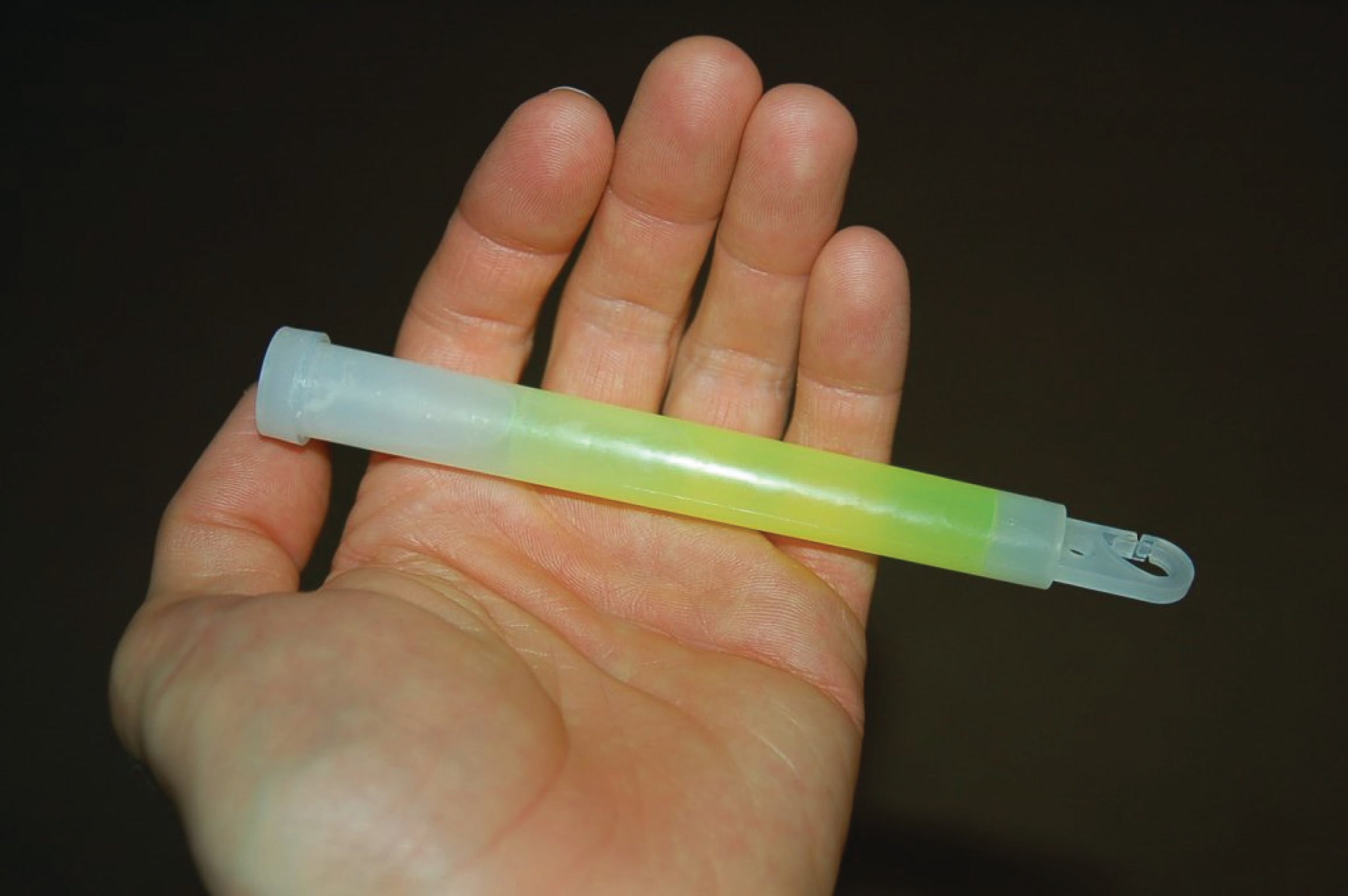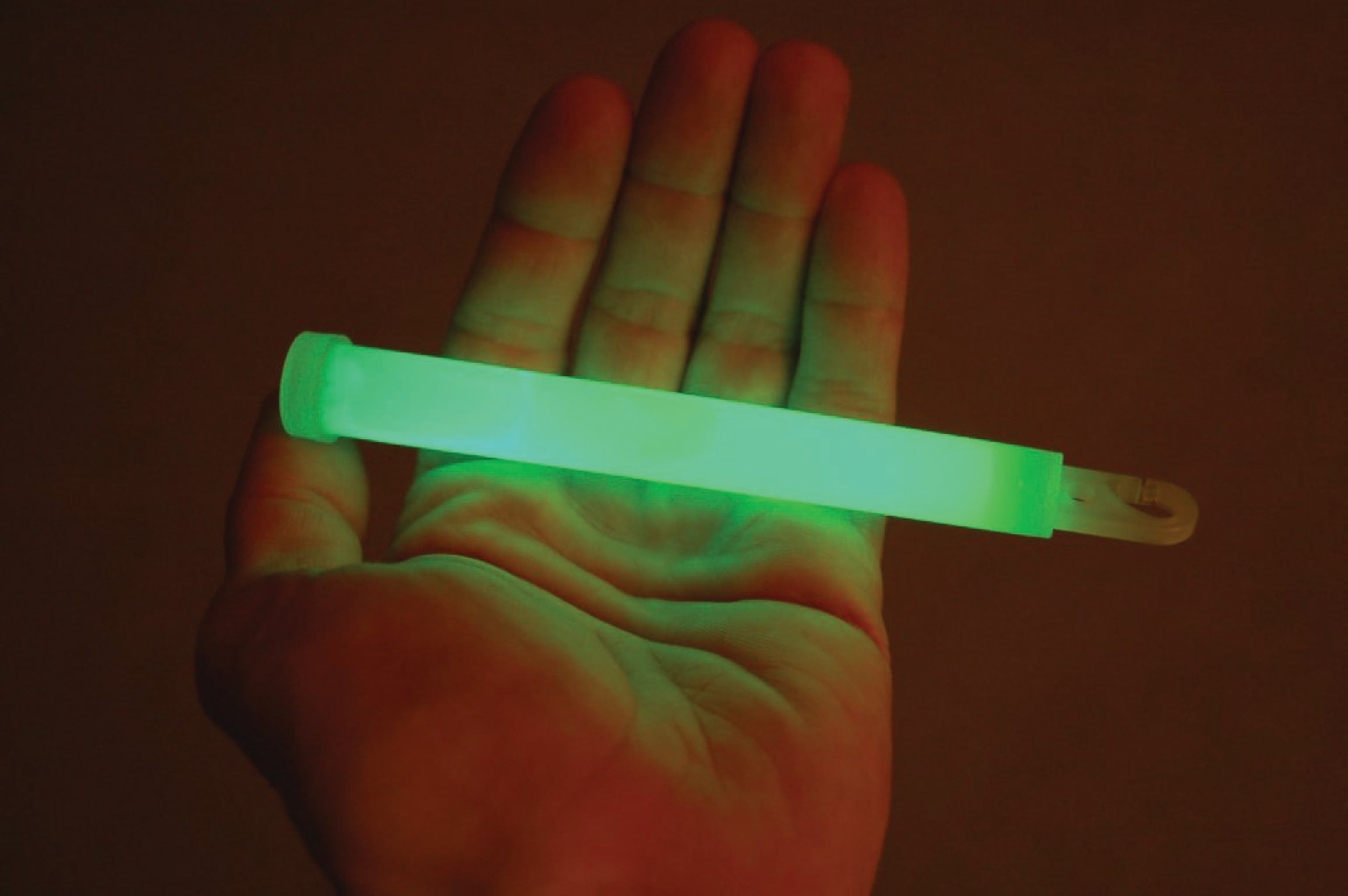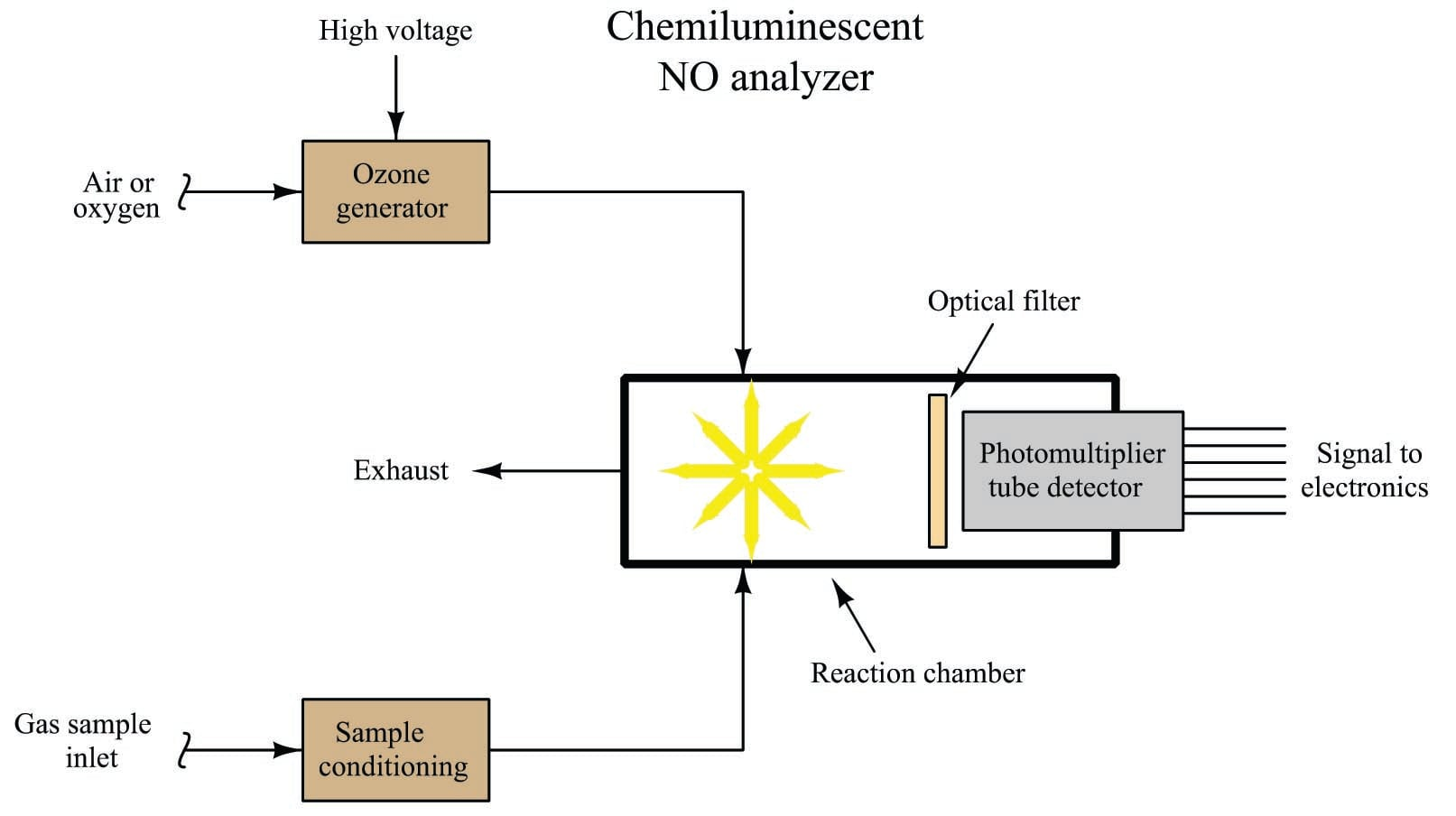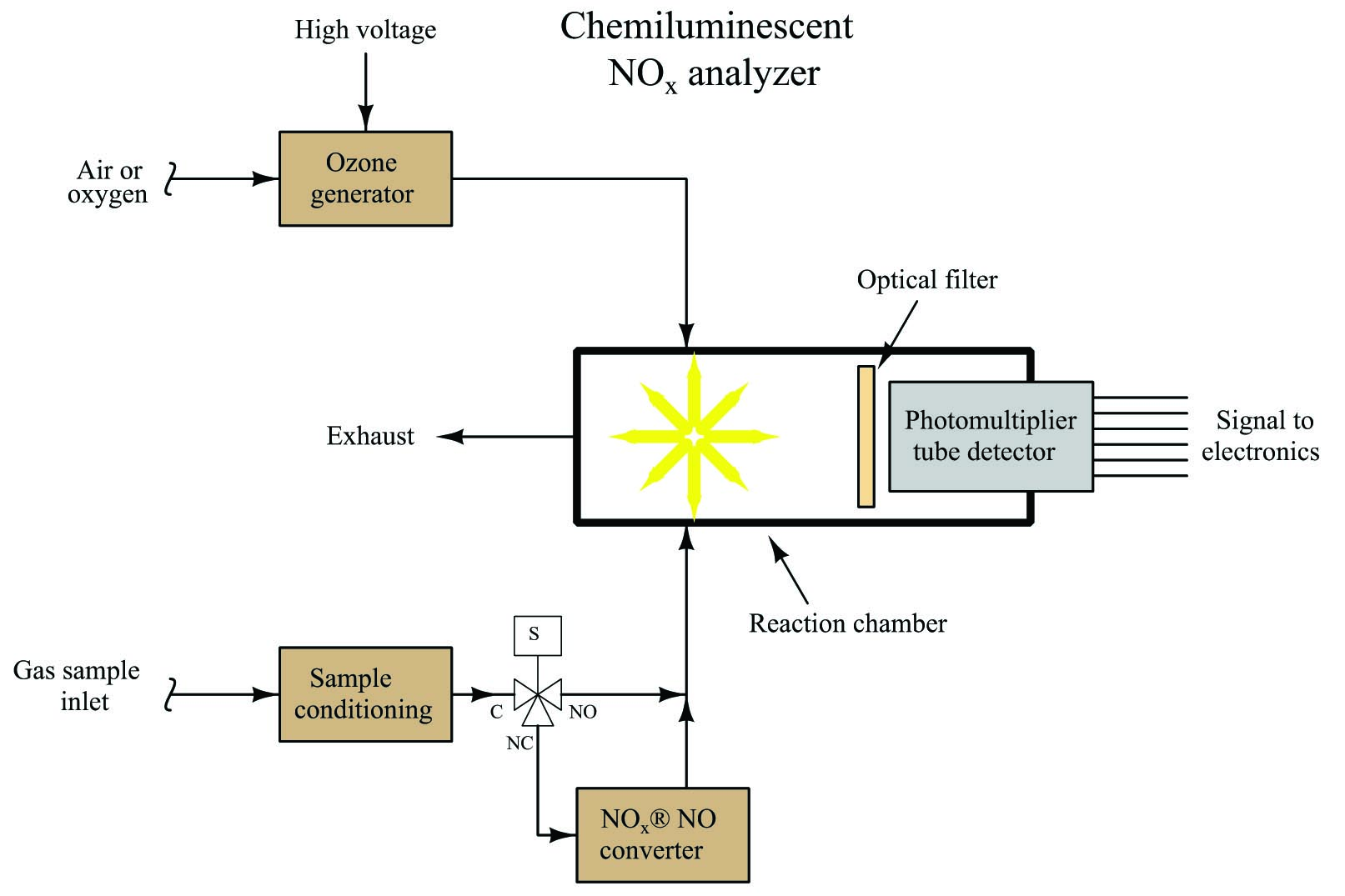Chemiluminescence Measurement

Chapter 23 - Introduction to Continuous Analytical Measurement

Recall that an exothermic chemical reaction is one that releases a net sum of energy, as opposed to an endothermic reaction which requires a greater input of energy than it releases. Combustion is a common class of exothermic reactions, with the released energy being very obviously in the forms of heat and light, with heat being the predominant form.

Some exothermic reactions release energy primarily in the form of light rather than heat. The general term for this effect is chemiluminescence. A natural example is the “cold” light emitted by North American species of firefly. In this small insect, a chemical reaction intermittently takes place emitting significant amounts of light but insignificant amounts of heat. An artificial example is the light emitted by a “glow stick” when activated. The following photographs show such a light source before activation when the reactants are separated (left) and after activation when the internal barrier is broken and the reactants are allowed to mix (right):Certain industrial compounds engage in chemiluminescent reactions, and this phenomenon may be used to measure the concentration of those compounds. One such compound is nitric oxide (NO), an atmospheric pollutant formed by high-temperature combustion with air as the oxidizer.

A spontaneous chemical reaction between nitric oxide and ozone (an unstable molecule formed of three oxygen atoms: O$$_{3}$$) is known to produce chemiluminescence:

$\hbox{NO} + \hbox{O}_3 \to \hbox{NO}_2 + \hbox{O}_2 + \hbox{ light}$

Although this process of generating light is quite inefficient (only a small fraction of the NO$$_{2}$$ molecules formed by this reaction will emit light), it is predictable enough to be used as a quantitative measurement method for nitric oxide gas. Ozone gas is very easy to produce on-demand, by exposing air or oxygen to a high-voltage electric discharge.

A simplified diagram for a chemiluminescent nitric oxide gas analyzer appears here:As with many optical analyzers, a photomultiplier tube serves as the light-detecting sensor, generating an electrical signal in proportion to the amount of light observed inside the reaction chamber. The higher the concentration of NO molecules in the sample gas stream, the more light will be emitted inside the reaction chamber, resulting in a stronger electrical signal produced by the photomultiplier tube.

Although this instrument readily measures the concentration of nitric oxide (NO), it is insensitive to other oxides of nitrogen (NO$$_{2}$$, NO$$_{3}$$, etc., collectively referred to as NO$$_{x}$$, pronounced “nocks”). Normally, we would consider this selectivity to be a good thing because it would eliminate interference problems from these other gases. However, as it so happens, these other oxides of nitrogen are every bit as polluting as nitric oxide, and therefore when we measure nitric oxide for pollution monitoring purposes, we usually also wish to measure these other oxides in combination.

In order to use chemiluminescence to measure all oxides of nitrogen, we must chemically convert the other oxides into nitric oxide (NO) before the sample enters the reaction chamber. This is done in a special module of the analyzer called a converter:A three-way solenoid valve is shown in this diagram, providing a means to bypass the converter so the analyzer only measures nitric oxide content in the sample gas. With the solenoid valve passing all the sample through the converter, the analyzer responds to all oxides of nitrogen (NO$$_{x}$$) and not just nitric oxide (NO).

One simple way to achieve the NO$$_{x}$$ $$\to$$ NO chemical conversion is to simply heat the sample gas to a high temperature, around 1300 $$^{o}$$F. At this temperature, the molecular structure of NO is favored over more complex oxides such as NO$$_{2}$$, the result being a release of oxygen from the NO$$_{2}$$ and NO$$_{3}$$ molecules to become NO molecules. A disadvantage of this technique is that those same high temperatures also have a tendency to convert other compounds of nitrogen such as ammonia (NH$$_{3}$$) into nitric oxide, thereby creating an unintended interference species.

An alternative NO$$_{x}$$ $$\to$$ NO conversion technique is to use a metallic reactant in the converter to remove the extra oxygen atoms from the NO$$_{2}$$ molecules. One such metal that works well for this purpose is molybdenum (Mo) heated to the comparatively low temperature of 750 $$^{o}$$F, which is too low to convert ammonia into nitric oxide. The reaction of NO$$_{2}$$ converting to NO is as follows:

$3\hbox{NO}_2 + \hbox{Mo} \to \hbox{MoO}_3 + 3\hbox{NO}$

Other oxides (such as NO$$_{3}$$) convert in a similar fashion, leaving their excess oxygen atoms bound to molybdenum atoms and becoming nitric oxide (NO). The only difference between these reactions and the one shown for NO$$_{2}$$ is the proportional (stoichiometric) ratios between molecules.

As you can see from the reaction, the molybdenum metal is converted into the compound molybdenum trioxide over time, requiring periodic replacement. The rate at which the molybdenum metal depletes inside the converter depends on the sample flow rate and the concentration of NO$$_{2}$$.

As with other optical gas analyzers, pressure control of the gas sample is critically important for good measurement accuracy. If the pressure of the sampled gas inside the chemiluminescence reaction chamber happens to vary, it will affect the amount of light emitted even if the relative concentration of NO$$_{x}$$ gas remains stable. This is because higher pressures will pack gas molecules closer together, resulting in more reactive molecules inside the chamber for any given percentage or ppm concentration. For this reason, you will see analyzers such as this equipped with pressure regulation to ensure the gas pressure inside the measurement chamber remains constant.

Published under the terms and conditions of the Creative Commons Attribution 4.0 International Public License